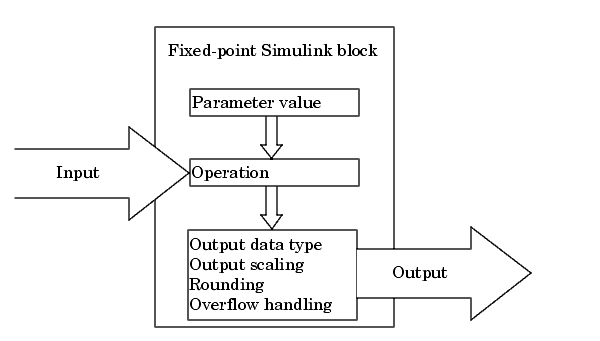## Parameter and Signal Conversions

### Introduction

To completely understand the results generated by fixed-point Simulink® blocks, you must be aware of these issues:

• When numerical block parameters are converted from doubles to fixed-point data types

• When input signals are converted from one fixed-point data type to another (if at all)

• When arithmetic operations on input signals and parameters are performed

For example, suppose a fixed-point Simulink block performs an arithmetic operation on its input signal and a parameter, and then generates output having characteristics that are specified by the block. The following diagram illustrates how these issues are related.The sections that follow describe parameter and signal conversions. Rules for Arithmetic Operations discusses arithmetic operations.

### Parameter Conversions

Parameters of fixed-point blocks that accept numerical values are always converted from `double` to a fixed-point data type. Parameters can be converted to the input data type, the output data type, or to a data type explicitly specified by the block. For example, the Discrete FIR Filter block converts its Initial states parameter to the input data type, and converts its Numerator coefficient parameter to a data type you explicitly specify via the block dialog box.

Parameters are always converted before any arithmetic operations are performed. Additionally, parameters are always converted offline using round-to-nearest and saturation. Offline conversions are discussed below.

Note

Because parameters of fixed-point blocks begin as `double`, they are never precise to more than 53 bits. Therefore, if the output of your fixed-point block is longer than 53 bits, your result might be less precise than you anticipated.

#### Offline Conversions

An offline conversion is a conversion performed by your development platform (for example, the processor on your PC), and not by the fixed-point processor you are targeting. For example, suppose you are using a PC to develop a program to run on a fixed-point processor, and you need the fixed-point processor to compute

`$y=\left(\frac{ab}{c}\right)u=Cu$`

over and over again. If a, b, and c are constant parameters, it is inefficient for the fixed-point processor to compute ab/c every time. Instead, the PC's processor should compute ab/c offline one time, and the fixed-point processor computes only C·u. This eliminates two costly fixed-point arithmetic operations.

### Signal Conversions

Consider the conversion of a real-world value from one fixed-point data type to another. Ideally, the values before and after the conversion are equal.

`${V}_{a}={V}_{b},$`

where Vb is the input value and Va is the output value. To see how the conversion is implemented, the two ideal values are replaced by the general [Slope Bias] encoding scheme described in Scaling:

`${V}_{i}={F}_{i}{2}^{{E}_{i}}{Q}_{i}+{B}_{i}.$`

Solving for the output data type's stored integer value, Qa is obtained:

`$\begin{array}{c}{Q}_{a}=\frac{{F}_{b}}{{F}_{a}}{2}^{{E}_{b}-{E}_{a}}{Q}_{b}+\frac{{B}_{b}-{B}_{a}}{{F}_{a}}{2}^{-{E}_{a}}\\ ={F}_{s}{2}^{{E}_{b}-{E}_{a}}{Q}_{b}+{B}_{net},\end{array}$`

where Fs is the adjusted fractional slope and Bnet is the net bias. The offline conversions and online conversions and operations are discussed below.

#### Offline Conversions

Both Fs and Bnet are computed offline using round-to-nearest and saturation. Bnet is then stored using the output data type and Fs is stored using an automatically selected data type.

#### Online Conversions and Operations

The remaining conversions and operations are performed online by the fixed-point processor, and depend on the slopes and biases for the input and output data types. The conversions and operations are given by these steps:

1. The initial value for Qa is given by the net bias, Bnet:

`${Q}_{a}={B}_{net}.$`
2. The input integer value, Qb, is multiplied by the adjusted slope, Fs:

`${Q}_{RawProduct}={F}_{s}{Q}_{b}.$`
3. The result of step 2 is converted to the modified output data type where the slope is one and bias is zero:

`${Q}_{Temp}=convert\left({Q}_{RawProduct}\right).$`

This conversion includes any necessary bit shifting, rounding, or overflow handling.

4. The summation operation is performed:

`${Q}_{a}={Q}_{Temp}+{Q}_{a}.$`

This summation includes any necessary overflow handling.

#### Streamlining Simulations and Generated Code

Note that the maximum number of conversions and operations is performed when the slopes and biases of the input signal and output signal differ (are mismatched). If the scaling of these signals is identical (matched), the number of operations is reduced from the worst (most inefficient) case. For example, when an input has the same fractional slope and bias as the output, only step 3 is required:

`${Q}_{a}=convert\left({Q}_{b}\right).$`

Exclusive use of binary-point-only scaling for both input signals and output signals is a common way to eliminate mismatched slopes and biases, and results in the most efficient simulations and generated code.

## SupportGet trial now